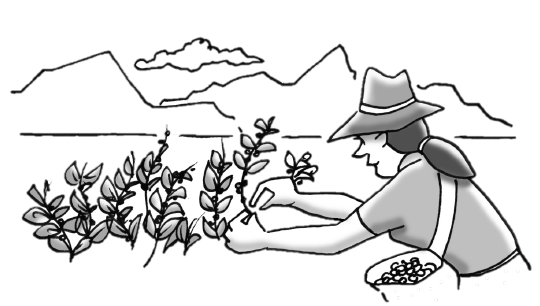### Home > CC2MN > Chapter 8 CC1: Unit 8 > Lesson CC1: 8.1.3 > Problem8-41

8-41.Angel is picking mountain blueberries for a delicious pie. She can pick $\frac { 1 } { 6 }$ cup of blueberries in $2$ minutes. If she needs $2\frac{1}{2}$ cups of blueberries for the pie, how long will it take her to pick the berries?

Set up a proportion, putting the rate in which she picks up blueberries over the time it takes her to do so.

Set up the proportion.

$\frac{\frac{1}{6}}{2}=\frac{\frac{5}{2}}{x}$

Cross-multiply and solve for $x$.

$\frac{1}{6} \cdot {x}=2 \cdot \frac{5}{2}$

$x=30$ minutes